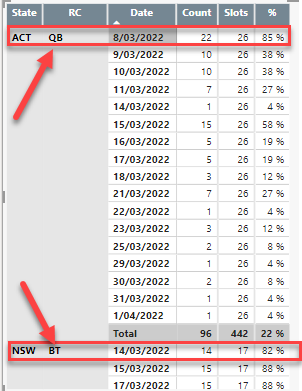cancel
Showing results for
Did you mean:

Fabric is Generally Available. Browse Fabric Presentations. Work towards your Fabric certification with the Cloud Skills Challenge.Anonymous
Not applicable

## First Date where the % is less than 90

Hi,

I have below report, I want to show the first date where the % is less than 90% per State per RC.Can anyone help?

2 ACCEPTED SOLUTIONSSuper User

Hi,

Please check the below picture and the attached pbix file.``````First date less than 90% =
VAR newtable =
SUMMARIZE ( Data, Data[State], Data[RC], Data[Date] ),
"@ratio", CALCULATE ( DIVIDE ( SUM ( Data[Count] ), SUM ( Data[Slots] ) ) )
)
VAR filtertable =
FILTER ( newtable, [@ratio] < 0.9 )
RETURN
IF ( HASONEVALUE ( Data[State] ), MINX ( filtertable, Data[Date] ) )
``````

If this post helps, then please consider accepting it as the solution to help other members find it faster, and give a big thumbs up.Anonymous
Not applicable

This is my DAX:

First date less than 90% =
VAR newtable =
SUMMARIZE ( Count, Location[State], Location[RC], Data[Date] ),
"@ratio", CALCULATE ( DIVIDE ( SUM ( Count[Count] ), SUM ( Slots[Slots] ) ) )
)
VAR filtertable =
FILTER ( newtable, [@ratio] < 0.9 )
RETURN
IF ( HASONEVALUE ( Location[State] ), MINX ( filtertable, Data[Date] ) )

and I get below, it correctly filter <90, but not the min date per RC:9 REPLIES 9Anonymous
Not applicable

Hi @Jihwan_Kim ,

It is working now, thanks for your help.Super User

Hi,

Please check the below picture and the attached pbix file.``````First date less than 90% =
VAR newtable =
SUMMARIZE ( Data, Data[State], Data[RC], Data[Date] ),
"@ratio", CALCULATE ( DIVIDE ( SUM ( Data[Count] ), SUM ( Data[Slots] ) ) )
)
VAR filtertable =
FILTER ( newtable, [@ratio] < 0.9 )
RETURN
IF ( HASONEVALUE ( Data[State] ), MINX ( filtertable, Data[Date] ) )
``````

If this post helps, then please consider accepting it as the solution to help other members find it faster, and give a big thumbs up.Anonymous
Not applicable

Hi @Jihwan_Kim ,

Just on last thing, Can you please help with how to get the Count and slots figure for the first date, at the moment I get the sum.

Thanks.Super User

Hi,

Thank you.

``````Count on First date less than 90% =
VAR newtable =
SUMMARIZE ( Data, Data[State], Data[RC], Data[Date], Data[Slots], Data[Count] ),
"@ratio", CALCULATE ( DIVIDE ( SUM ( Data[Count] ), SUM ( Data[Slots] ) ) )
)
VAR filtertable =
FILTER ( newtable, [@ratio] < 0.9 )
VAR mindate =
MINX ( filtertable, Data[Date] )
VAR countfigure =
SUMX ( FILTER ( newtable, Data[Date] = mindate ), Data[Count] )
RETURN
IF ( HASONEVALUE ( Data[State] ), countfigure )``````

``````Slots on First date less than 90% =
VAR newtable =
SUMMARIZE ( Data, Data[State], Data[RC], Data[Date], Data[Slots], Data[Count] ),
"@ratio", CALCULATE ( DIVIDE ( SUM ( Data[Count] ), SUM ( Data[Slots] ) ) )
)
VAR filtertable =
FILTER ( newtable, [@ratio] < 0.9 )
VAR mindate =
MINX ( filtertable, Data[Date] )
VAR slotsfigure =
SUMX ( FILTER ( newtable, Data[Date] = mindate ), Data[Slots] )
RETURN
IF ( HASONEVALUE ( Data[State] ), slotsfigure )``````

If this post helps, then please consider accepting it as the solution to help other members find it faster, and give a big thumbs up.Anonymous
Not applicable

Hi @Jihwan_Kim ,

I get error because the date is in Date table, State is in the Location table, they are not in the same table.Anonymous
Not applicable

Also the Count and Slots are in another different tables.Super User

HI,

Sorry that I cannot imagine how your data model looks like.

I think, sharing your sample pbix file will help.

If this post helps, then please consider accepting it as the solution to help other members find it faster, and give a big thumbs up.Anonymous
Not applicable

I can't share the pbix because I have tabular model.

Attached is a simple draw of the model.Anonymous
Not applicable

This is my DAX:

First date less than 90% =
VAR newtable =
SUMMARIZE ( Count, Location[State], Location[RC], Data[Date] ),
"@ratio", CALCULATE ( DIVIDE ( SUM ( Count[Count] ), SUM ( Slots[Slots] ) ) )
)
VAR filtertable =
FILTER ( newtable, [@ratio] < 0.9 )
RETURN
IF ( HASONEVALUE ( Location[State] ), MINX ( filtertable, Data[Date] ) )

and I get below, it correctly filter <90, but not the min date per RC:Announcements#### Power BI Monthly Update - November 2023

Check out the November 2023 Power BI update to learn about new features.#### Fabric Community News unified experience

Read the latest Fabric Community announcements, including updates on Power BI, Synapse, Data Factory and Data Activator.#### Exclusive opportunity for Women!

Join us for a free, hands-on Microsoft workshop led by women trainers for women where you will learn how to build a Dashboard in a Day!#### The largest Power BI and Fabric virtual conference

130+ sessions, 130+ speakers, Product managers, MVPs, and experts. All about Power BI and Fabric. Attend online or watch the recordings.Top Solution Authors
Top Kudoed Authors
Users online (3,066)